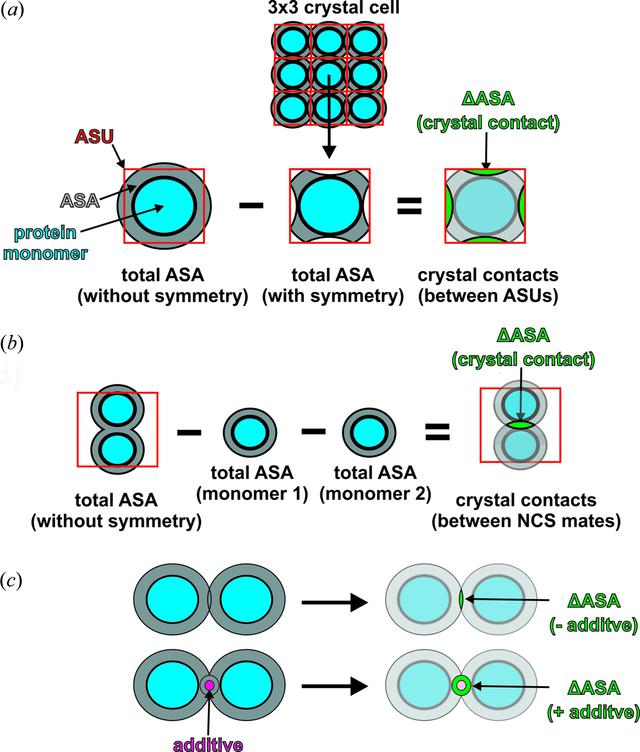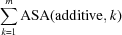disable zoom     view article Figure 1 Schematic overview of the ASA calculations. (a) Calculation of the ΔASA(ASU) of an ASU containing one single monomer according to equation (1). ΔASA(ASU) was obtained by subtracting the total overall ASA of the ASU considering the interactions between symmetry mates from different ASUs [ASA(+symmetry), ASU–ASU interactions are indicated by the 3 × 3 crystal cell, middle] from the total overall ASA of the ASU excluding symmetry-mate interactions [ASA(−symmetry), left]. The resulting crystal contacts, ΔASA(ASU), are indicated by the green areas (right). Additives have been omitted for clarity. (b) Calculation of the ΔASA within the ASU containing two monomers. ΔASA(within ASU) was calculated using equation (2), namely by subtracting the total overall ASA of every monomer [ASA(monomer), middle] from the overall ASA excluding crystal packing but including interactions between NCS mates [ASA(−symmetry), left]. The resulting crystal contacts between NCS mates within the ASA are shown in green (right). Additives have been omitted for clarity and therefore the additive term,, of equation (2)has to be ignored for this graphic. To obtain the total ΔASA, ΔASA(total), for structures possessing more than one monomer in the ASU, the results of equations (1) (part a) and (2) (part b) had to be summed as shown in equation (3). (c) Possible impact of an additive on ΔASA as the crystal contact increases in the presence of the additive (bottom) compared to the same situation without the additive (top). The resulting difference areas are colored green.

IUCrJ
Volume 4| Part 6| November 2017| Pages 734-740
ISSN: 2052-2525